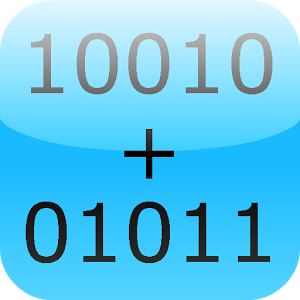\$0.99

# Android Giveaway of the Day - Binary Calculator Pro

This app is the pro version of Binary Calculator
\$0.99 期限切れ
ユーザーの評価: 3 (50%) 3 (50%)

このgiveawayオファーはすでに期限が切れています。Binary Calculator Proが今紹介されています。

This app is the pro version of Binary Calculator. Completely without advertisement!

This is a free mathematical calculator, which is able to add, subtract, multiply and divide numbers in binary format (used in computers).

Any number can be represented by any sequence of bits (binary digits), they are commonly written using the symbols 0 and 1.

Best math tool for school and college! If you are a student, it will helps you to learn arithmetics for computer science and electrical engineering.

GK Apps

Education

4.0

Everyone

1.6 and up

## コメント Binary Calculator Pro

Thank you for voting!